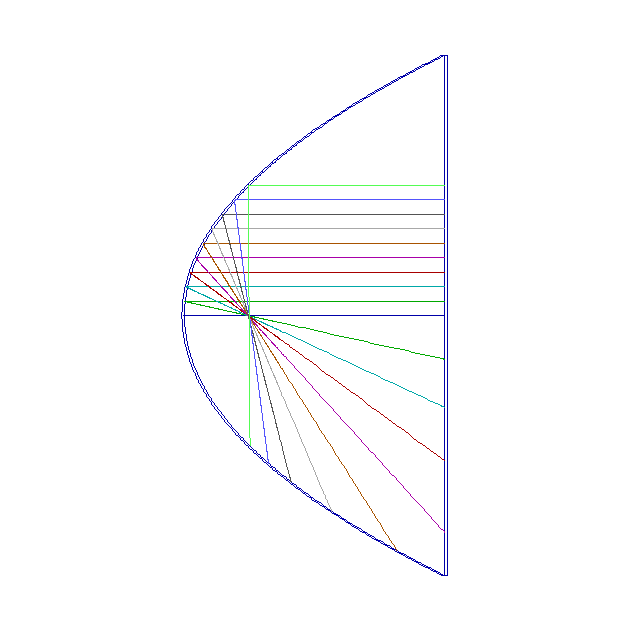CAPABILITIES
USER DEFINED EQUATIONS OPTION

### Allows users to enter their own equations to define electrode shapes -a powerful option and unique in this field, as far as we know.

Some of the benchmark and example files that illustrate this option are test2d27 and test3d33 for parabolic mirrors, test3d34 and xmpl3d95 for quadrupole ion traps, and shap2d05 for a hyperbolic electrode.

The figure below shows a simple 2D parabolic mirror (from test2d27).  The 2 parabolic electrodes are at voltages 0 and -2 and so electrons of energy 1eV are reflected between to create a mirror.  The incident parallel beam is correctly focussed to a point.It is extremely easy to set up such a surface.  Here the inner parabola has the equation z = r^2, which the user puts into the databuilder.  After the user has specified the number of subdivisions etc, the program does the rest.  A wide range of operators and functions can be used in the equations, which can have a length up to 50 characters.

expand_less